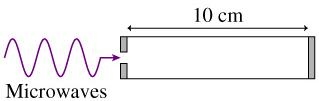# Problem: A microwave generator can produce microwaves at any frequency between 10.0 GHz and 20.0 GHz. As the figure shows, the microwaves are aimed, through a small hole, into a "microwave cavity" that consists of a 10.0 cm-long cylinder with reflective ends.Part A. Which frequencies between 10 GHz and 20 GHz will create standing waves in the microwave cavity?Enter your answers numerically in increasing order separated by commas.f = _________________________ GHzPart BFor which of these frequencies is the cavity midpoint an antinode?Enter your answers numerically in increasing order separated by commas.fa = ___________________________ GHz

###### FREE Expert Solution

Since two ends of the microwave cavity are reflective, we'll assume that it is a closed pipe.

The wavelength that forms standing waves is expressed as:

$\overline{){\mathbf{\lambda }}{\mathbf{=}}\frac{\mathbf{2}\mathbf{L}}{\mathbf{n}}}$, where λ is the wavelength forming standing waves, L is the length of the cavity, and n is the order.

The frequency of the wave is:

$\overline{){\mathbf{f}}{\mathbf{=}}\frac{\mathbf{c}}{\mathbf{\lambda }}}$, where c is the speed of light

Part A

We get the frequencies that will create standing waves in the microwave cavity by substituting λ = 2L/n in f = c/λ.

So, we have:

$\overline{)\begin{array}{rcl}{\mathbf{f}}& {\mathbf{=}}& \frac{\mathbf{c}}{\mathbf{\left(}\frac{\mathbf{2}\mathbf{L}}{\mathbf{n}}\mathbf{\right)}}\\ & {\mathbf{=}}& \frac{\mathbf{cn}}{\mathbf{2}\mathbf{L}}\end{array}}$

In the first case, the standing wave will be formed when n = 7.

83% (102 ratings)###### Problem Details

A microwave generator can produce microwaves at any frequency between 10.0 GHz and 20.0 GHz. As the figure shows, the microwaves are aimed, through a small hole, into a "microwave cavity" that consists of a 10.0 cm-long cylinder with reflective ends.Part A. Which frequencies between 10 GHz and 20 GHz will create standing waves in the microwave cavity?
Enter your answers numerically in increasing order separated by commas.
f = _________________________ GHz

Part B
For which of these frequencies is the cavity midpoint an antinode?
Enter your answers numerically in increasing order separated by commas.
fa = ___________________________ GHz

Frequently Asked Questions

What scientific concept do you need to know in order to solve this problem?

Our tutors have indicated that to solve this problem you will need to apply the Standing Waves concept. You can view video lessons to learn Standing Waves. Or if you need more Standing Waves practice, you can also practice Standing Waves practice problems.